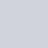1.2.3.4."
"

# Understanding How Many Centimeters Is an Inch with Examples?How many centimeters is an inch? Provided that 1 inch is equivalent to 2.54 centimeters, multiply the given amount by 2.54. See how many centimeters an inch.

## How Many Centimeters Are in an Inch- Inch to Cm Conversion?

The units used to measure length include meters, centimeters, feet, and inches. We frequently encounter circumstances while measuring length where there are numerous units of measurement available. To get the desired result in such circumstances, it is necessary to balance many units within a single unit. When dealing with length-related math problems, one such conversion that we perform is from inches to centimeters. How many Centimeters is an Inch? Since 1 inch is equal to 2.54 centimeters, multiply the above number by 2.54 to obtain your answer or convert it from inches to centimeters.

## Cm to Inch Conversion

The centimeter and the inch are both length units. The abbreviation for an inch is “in,” whereas the abbreviation for a centimeter is “cm.”

Fact:

There is 2.54 cm in one inch.

0.3937 inches is equal to 1 centimeter.

Step 1: To transform the supplied amount from inches to centimeters, multiply it by 2.54 and change the unit from “in” to “cm.” At this point, the value has been changed from inches to centimeters.

Step 2: Now that the value has been converted from centimeters to inches, multiply it by 0.3937 and change the unit from “cm” to “in.”

Example: how much are 6 inches in centimeters?

2.4 centimeters make up one inch.

We need to translate this amount from inches to centimeters, so we’ll multiply it by 2.54 to get the new measurement.

= 6*2.54 = 15.24 cm

Consequently, 6 inches is 15.24 cm.

Example: Convert 8 cm to inches?

Solution: Given 8 cm

Knowing that 1 cm equals 0.3937 inches

Here are value is in centimeters and we need to convert it to inches, so we’ll multiply our value by 0.3937 to convert it to inches.

= 8*0.3937 = 3.14 inches

So 8 centimeters is equal to 3.14 inches.

## Inch-to-Centimeter Chart

Here we have given the Inch to Centimeter chart, where we have converted the various values of Inches to Centimeters. See the full table for inch-to-centimeter conversion practice and try converting all of these values. Take a look at the table given below:

 Inches (in) Centimeters (cm) 1 inch 2.54 cm 2 inches 5.08 cm 3 inches 7.62 cm 4 inches 10.16 cm 5 inches 12.70 cm 6 inches 15.24 cm 7 inches 17.78 cm 8 inches 20.32 cm 9 inches 22.86 cm 10 inches 25.40 cm 50 inches 127.00 cm 100 inches 254.00 cm

### Inches to Centimeters (Cm) Conversion Example

Example 1: Convert 20 inches to centimeters.

Solution: Given what we already know 1 inch = 2.54 cm.

So, to convert 20 inches to centimeters

we have to multiply 20 by 2.54

Hence, 20 inches = 20 × 2.54 cm = 50.8 cm.

So 20 inches is equal to 50.8 centimeters.

Example 2: Convert 12 inches to centimeters.

Solution: Given what we already know 1 inch = 2.54 cm.

So, to convert 12 inches to centimeters

we have to multiply 12 by 2.54

Hence, 12 inches = 12 × 2.54 cm = 30.48 cm.

Hence 12 inches is equal to 30.48 centimeters.

"
"